Courses
Courses for Kids
Free study material
Free LIVE classes
More

# NCERT Solutions for Class 8 Maths Chapter 10 - ExerciseLIVE
Join Vedantu’s FREE Mastercalss

## NCERT Solutions for Class 8 Maths Chapter 10 Visualising Solid Shapes (EX 10.3) Exercise 10.3

Free PDF download of NCERT Solutions for Class 8 Maths Chapter 10 Exercise 10.3 (EX 10.3) and all chapter exercises at one place prepared by expert teacher as per NCERT (CBSE) books guidelines. Class 8 Maths Chapter 10 Visualising Solid Shapes Exercise 10.3 Questions with Solutions to help you to revise complete Syllabus and Score More marks. Register and get all exercise solutions in your emails.

Vedantu is a platform that provides free NCERT Solution and other study materials for students. Science Students who are looking for NCERT Solutions for Class 8 Science will also find the Solutions curated by our Master Teachers really Helpful.

Last updated date: 22nd Sep 2023
Total views: 508.5k
Views today: 14.08k

## Access NCERT Solution for Class 8 Maths Chapter 10 – Visualising Solid Shapes

Exercise 10.3

Refer to pages 14-17 for exercise 10.3 in the PDF

1. Can a polyhedron have for its faces

(i) 3 triangles?

Ans: A polyhedron with 3 triangular faces is not possible. Because the edges of a polyhedron must meet at vertices. A polyhedron has a minimum of 4 faces.

(ii) 4 triangles?

Ans: Yes, a triangular pyramid has 4 triangular faces. Because all the eight edges meet at the vertices(iii) a square and four triangles?

Ans: Yes, a square pyramid has a square face and 4 triangular faces. Because all the eight edges meet at the vertices.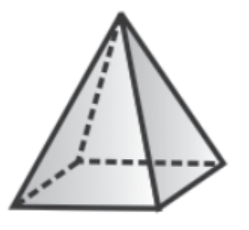2. Is it possible to have a polyhedron with any given number of faces? (Hint: Think of a pyramid).

Ans: A polyhedron has a minimum of 4 faces.

3. Which are prisms among the following?

(i) A nail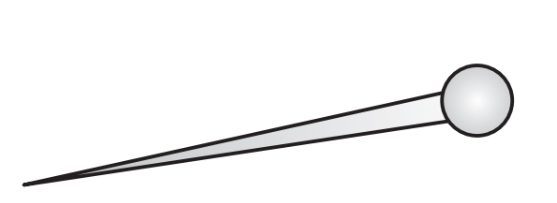Ans: It is not a prism.It is not a polyhedron as it has a curved surface.

(ii) Unsharpened pencil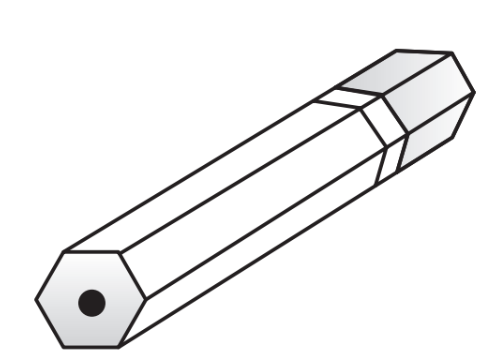Ans: It is a prism.

(iii) A table weight

(Image Will Be Updated Soon)

Ans: It is not a prism. It is a pyramid.

(iv) A box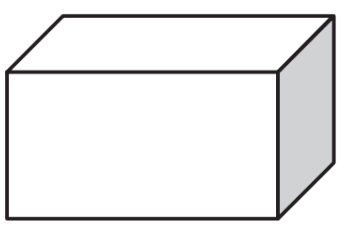Ans: It is a prism.

4. (i) How are prisms and cylinders alike?

Ans: A cylinder is a prism that has a circle as its base. It is called a circular prism.

(ii) How are pyramids and cones alike?

Ans: A cone is a pyramid that has a circle as its base. So it can be called a circular pyramid.

5. Is a square prism the same as a cube? Explain.

Ans: A square prism has a square as its base. However, its height is not necessarily sameas the side of the square. Thus, a square prism can also be a cuboid.

6. Verify Euler’s formula for these solids.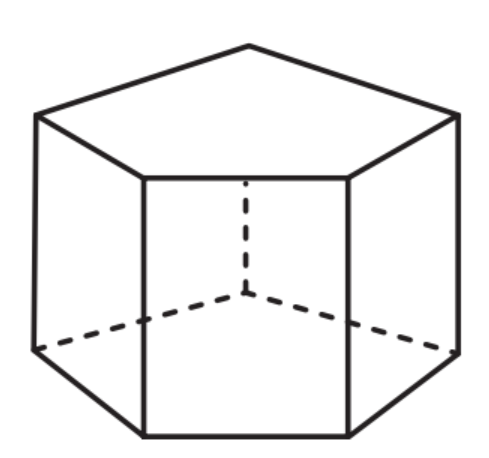(i)

Ans: By Euler’s formula, we have

\[F + V - E = 2\]

Here \[F\], \[V\] and \[E\] are the number of faces ,vertices and edges respectively.

In first figure,

Number of faces,\[F = 7\]

Number of vertices,\[V = 10\]

Number of edges ,\[E = 15\]

We have, \[F + V - E = 7 + 10 -15\]\[ = 17 - 15 = 2\]

Hence, Euler’s formula is verified.

(ii)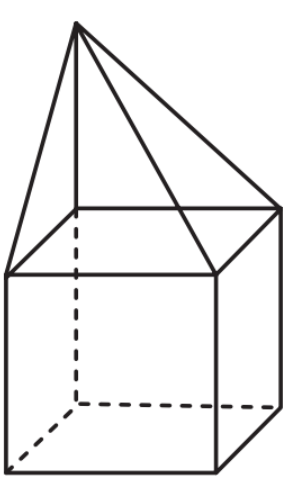Ans: Number of faces \[F = 9\]

Number of vertices, \[V = 9\]

Number of edges, \[E = 16\]

We have, \[F + V - E = 9 + 9 -16\]\[ = 18 - 16 = 2\]

Hence, Euler’s formula is verified.

7. Using Euler’s formula, find the unknown.

 Faces ? 5 20 Vertices 6 ? 12 Edges 12 9 ?

Ans: By Euler’s formula, we have

\[F + V - E = 2\]

(i) Ans: Number of vertices, \[V = 5\]

Number of edges, \[E = 12\]

Then substitute the given values in Euler’s formula

\[F + 6 - 12 = 2\]

\[F - 6 = 2\]

\[F = 8\]

(ii) Ans: Number of faces, \[F = 5\]

Number of edges, \[E = 9\]

Then substitute the given values in Euler’s formula

\[5 + V - 9 = 2\]

\[V - 4 = 2\]

\[V = 6\]

(iii) Ans: Number of faces \[F = 20\]

Number of vertices, \[V = 12\]

Then substitute the given values in Euler’s formula

\[20 + 12 - E = 2\]

\[32 - E = 2\]

\[E = 30\]

Thus, the table can be completed as

 Faces 8 5 20 Vertices 6 6 12 Edges 12 9 30

8. Can a polyhedron have 10 faces, 20 edges and 15 vertices?

Ans: Number of faces, \[F = 10\]

Number of edges, \[E = 20\]

Number of vertices, \[V = 15\]

Polyhedron will satisfy the Euler’s Formula\[F + V - E = 2\]

For the given polygon,

\[F + V - E = 10 + 15 - 20\]

\[ = 25 - 20 = 5 \ne 2\]It is not satisfying Euler’s formula, such a polyhedron is not possible.

## NCERT Solutions for Class 8 Maths Chapter 10 Visualising Solid Shapes Exercise 10.3

Opting for the NCERT solutions for Ex 10.3 Class 8 Maths is considered as the best option for the CBSE students when it comes to exam preparation. This chapter consists of many exercises. Out of which we have provided the Exercise 10.3 Class 8 Maths NCERT solutions on this page in PDF format. You can download this solution as per your convenience or you can study it directly from our website/ app online.

Vedantu in-house subject matter experts have solved the problems/ questions from the exercise with the utmost care and by following all the guidelines by CBSE. Class 8 students who are thorough with all the concepts from the Maths textbook and quite well-versed with all the problems from the exercises given in it, then any student can easily score the highest possible marks in the final exam. With the help of this Class 8 Maths Chapter 10 Exercise 10.3 solutions, students can easily understand the pattern of questions that can be asked in the exam from this chapter and also learn the marks weightage of the chapter. So that they can prepare themselves accordingly for the final exam.

Besides these NCERT solutions for Class 8 Maths Chapter 10 Exercise 10.3, there are plenty of exercises in this chapter which contain innumerable questions as well. All these questions are solved/answered by our in-house subject experts as mentioned earlier. Hence all of these are bound to be of superior quality and anyone can refer to these during the time of exam preparation. In order to score the best possible marks in the class, it is really important to understand all the concepts of the textbooks and solve the problems from the exercises given next to it.

Do not delay any more. Download the NCERT solutions for Class 8 Maths Chapter 10 Exercise 10.3 from Vedantu website now for better exam preparation. If you have the Vedantu app in your phone, you can download the same through the app as well. The best part of these solutions is these can be accessed both online and offline as well.